# Colleges with the lowest SAT scores in New Hampshire

Top 8 colleges in New Hampshire with the lowest SAT scores
Looking for the colleges with the lowest SAT scores in New Hampshire? Well you're in luck! We've compiled a national college database and have created a list of the top 8 universities with the lowest SAT scores in New Hampshire below. If you are not a good test taker or worried about your test scores, this list is for you. These are the schools whose applicants had the lowest average SAT scores in New Hampshire, which means that you can get into these colleges with a lower SAT score. We also include each college's ACT scores and acceptance rate so that you can see where you would have the easiest time getting in. Read on to find out more.

## New England College SAT scores

The average SAT score for New England College is 1030.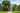The average SAT score of 1030 breaks down into:

• SAT math: 510

The average ACT score for New England College is 20 and their acceptance rate is 99.9%.

## Keene State College SAT scores

The average SAT score for Keene State College is 1040.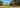The average SAT score of 1040 breaks down into:

• SAT math: 510

The average ACT score for Keene State College is 21 and their acceptance rate is 82.7%.

## Rivier University SAT scores

The average SAT score for Rivier University is 1044.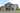The average SAT score of 1044 breaks down into:

• SAT math: 520

The average ACT score for Rivier University is 21 and their acceptance rate is 68.1%.

## Plymouth State University SAT scores

The average SAT score for Plymouth State University is 1070.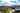The average SAT score of 1070 breaks down into:

• SAT math: 530

The average ACT score for Plymouth State University is 21 and their acceptance rate is 78.8%.

## Southern New Hampshire University SAT scores

The average SAT score for Southern New Hampshire University is 1070.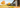The average SAT score of 1070 breaks down into:

• SAT math: 530

The average ACT score for Southern New Hampshire University is 22 and their acceptance rate is 92.9%.

## University of New Hampshire SAT scores

The average SAT score for University of New Hampshire is 1183.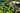The average SAT score of 1183 breaks down into:

• SAT math: 590

The average ACT score for University of New Hampshire is 25 and their acceptance rate is 76.8%.

## Saint Anselm College SAT scores

The average SAT score for Saint Anselm College is 1220.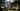The average SAT score of 1220 breaks down into:

• SAT math: 600

The average ACT score for Saint Anselm College is 26 and their acceptance rate is 76.6%.

## Dartmouth College SAT scores

The average SAT score for Dartmouth College is 1500.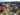The average SAT score of 1500 breaks down into:

• SAT math: 760